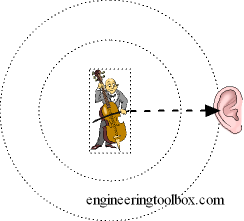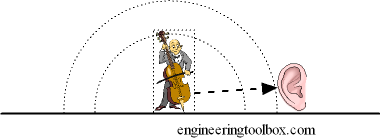Engineering ToolBox - Resources, Tools and Basic Information for Engineering and Design of Technical Applications!

# Sound - Abatement vs. the Distance from Source

## The disruption of the sound pressure wave and the reduction of noise is called attenuation - Sound Pressure Level vs. distance calculator.

The sound pressure from a source is reduced with distance from source.

### Spherical DistanceSound pressure in spherical distance from a noise source can be calculated as:

p2 = ρ c N / (4 π r2)              (1)

where

p = sound pressure (Pa, N/m2)

ρ = density of air (kg/m3)

c = speed of sound (m/s)

N = sound power (W)

π = 3.14

r = distance from source (m)

### Half Spherical DistanceSound pressure in half spherical distance from a source can be expressed as:

p2 = ρ c N / (4 π r2 / 2)

= 2 ρ c N / (4 π r2)                (2)

A more generic expression for sound pressure in distance from source can be formulated to:

p2 = D ρ c N / (4 π r2)              (3)

where

D = directivity coefficient (1 spherical, 2 half spherical)

The directivity coefficient depends on several parameters - the position and direction of the source, the room and the surrounding area, etc.

The Sound Pressure Level - Lp - can be expressed logarithmic in decibels as:

Lp = 20 log (p / pref)

= 20 log ((D ρ c N / (4 π r2))1/2 / pref)

= 20 log (1 / r (D ρ c N / (4 π))1/2 / pref)                 (4)

where

Lp = sound pressure level (dB)

pref = 2 10-5 - reference sound pressure (Pa)

Note! - a doubling of the distance from a sound source - will reduce the sound pressure level - Lp - with 6 decibels.

### Sound Pressure Level Calculator

ρ - air density (kg/m3)

c - sound velocity (m/s)

N - sound power (W)

r - distance from source (m)

### Example - Sound Pressure from a Wood Planer

The sound power generated from a wood planer is estimated to 0.01 W. The sound pressure in distance 10 m from the planner can be calculated as

Lp = 20 log ((D ρ c N / (4 π r2))1/2 / pref )

= 20 log (2 (1 kg/m3) (331.2 m/s) (0.01 W) / (4 π (10 m)2))1/2 / (2 10-5 Pa))

= 71 dB

## Related Topics

• ### Acoustics

Room acoustics and acoustic properties, decibel A, B and C, Noise Rating (NR) curves, sound transmission, sound pressure, sound intensity and sound attenuation.

## Related Documents

• ### Propagation of Sound Outdoors - Attenuation vs. Distance

The propagation of outdoors sound vs. distance and attenuation.
• ### Required Voice Level at Distance

Distance and perception of voice.
• ### Sound - Attenuation and the Directivity Coefficient

The attenuation in a room depends on the location of the sound source and the receiver - and the room constant.
• ### Sound Intensity

Acoustic power of sound per unit area.
• ### Sound Power

Sound power from sources like fans, jet engines, cars, humans and more.
• ### Sound Propagation - the Inverse Square Law

Doubling of the distance from a noise source reduces the sound pressure level with 6 decibel.
• ### Speed of Sound Equations

Calculate the speed of sound (the sonic velocity) in gases, fluids or solids.

## Engineering ToolBox - SketchUp Extension - Online 3D modeling!

Add standard and customized parametric components - like flange beams, lumbers, piping, stairs and more - to your Sketchup model with the Engineering ToolBox - SketchUp Extension - enabled for use with older versions of the amazing SketchUp Make and the newer "up to date" SketchUp Pro . Add the Engineering ToolBox extension to your SketchUp Make/Pro from the Extension Warehouse !

We don't collect information from our users. More about

## Citation

• The Engineering ToolBox (2005). Sound - Abatement vs. the Distance from Source . [online] Available at: https://www.engineeringtoolbox.com/abatement-distance-source-d_723.html [Accessed Day Month Year].

Modify the access date according your visit.

9.19.12